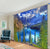Rs. 1,899.00
Rs. 3,800.00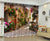Rs. 1,899.00
Rs. 3,800.00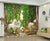Rs. 1,899.00
Rs. 3,800.00Rs. 1,899.00
Rs. 3,800.00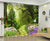Rs. 1,899.00
Rs. 3,800.00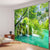Rs. 1,899.00
Rs. 3,800.00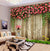Rs. 1,899.00
Rs. 3,800.00Rs. 1,899.00
Rs. 3,800.00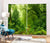Rs. 1,899.00
Rs. 3,800.00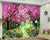Rs. 1,899.00
Rs. 3,800.00Rs. 1,899.00
Rs. 3,800.00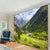Rs. 1,899.00
Rs. 3,800.00Rs. 1,899.00
Rs. 3,800.00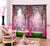Rs. 1,899.00
Rs. 3,800.00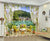Rs. 1,899.00
Rs. 3,800.00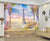Rs. 1,899.00
Rs. 3,800.00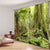Rs. 1,899.00
Rs. 3,800.00Rs. 1,899.00
Rs. 3,800.00Rs. 1,899.00
Rs. 3,800.00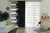Rs. 1,899.00
Rs. 3,800.00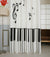Rs. 1,899.00
Rs. 3,800.00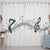Rs. 1,899.00
Rs. 3,800.00Rs. 1,899.00
Rs. 3,800.00Rs. 1,899.00
Rs. 3,800.00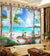Rs. 1,899.00
Rs. 3,800.00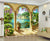Rs. 1,899.00
Rs. 3,800.00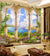Rs. 1,899.00
Rs. 3,800.00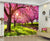Rs. 1,899.00
Rs. 3,800.00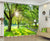Rs. 1,899.00
Rs. 3,800.00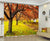Rs. 1,899.00
Rs. 3,800.00Rs. 1,899.00
Rs. 3,800.00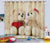Rs. 1,899.00
Rs. 3,800.00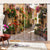Rs. 1,899.00
Rs. 3,800.00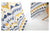Rs. 1,899.00
Rs. 3,800.00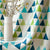Rs. 1,899.00
Rs. 3,800.00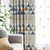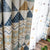Rs. 1,899.00
Rs. 3,800.00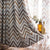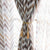Rs. 1,899.00
Rs. 3,800.00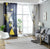Rs. 1,899.00
Rs. 3,800.00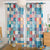Rs. 1,899.00
Rs. 3,800.00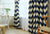Rs. 1,899.00
Rs. 3,800.00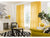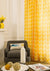Rs. 1,899.00
Rs. 3,800.00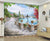Rs. 1,899.00
Rs. 3,800.00Rs. 1,999.00
Rs. 4,000.00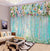Rs. 1,899.00
Rs. 3,800.00Rs. 1,999.00
Rs. 4,000.00

Free Delivery

100% Satisfaction Guarantee

Save & Secure Checkout